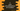# C program to print all combinations of three numbers# Introduction :

In this C programming tutorial, we will learn how to print all combinations of three predefined numbers. The program will take the numbers as user input and print out all different combinations.

## Algorithm :

The main idea behind the solution to this problem is to find out the combination of 0,1 and 3. First of all, we will put the user-provided numbers in an array. Then using three for loops one inside another, we will find out the different combinations of 0,1 and 3. We will consider these values as the index of the array. That will help us to print out the combination of the three user given numbers. The algorithm will look like below :

1. Take the numbers from the user.
2. Store the numbers in an array of size 3.
3. Using three for loops, find out all combinations of 0,1 and 3.
4. Whenever a new combination is found, print out the content of the array for that specific index for each loop.

## C program :

The c program will look like as below :

``````#include <stdio.h>

int main()
{
//1
int i, j, k;
int arr;
//2
printf("Enter the first number : ");
scanf("%d", &arr);
printf("Enter the second number : ");
scanf("%d", &arr);
printf("Enter the third number : ");
scanf("%d", &arr);
//3
for (i = 0; i < 3; i++)
{
for (j = 0; j < 3; j++)
{
for (k = 0; k < 3; k++)
{
if (i != j && j != k && k != i)
{
//4
printf("[%d %d %d]\n", arr[i], arr[j], arr[k]);
}
}
}
}
}``````

### Explanation :

The commented numbers in the above program denote the step numbers below :

1. Create three integers i,j and k to use in the for loops. Also, create one integer array of size 3.
2. Ask the user to enter all three numbers one by one. Read them and store them in the array on index _0,1 and 2 _respectively.
3. Run three for loops one inside another. Each loop will run from 0 to 2.
4. Inside the loops, check if the current value of each index of the loops is equal or not. If all are different, print out the numbers stored in the array for all specific index positions.

### Sample Output :

``````Enter the first number : 1
Enter the second number : 2
Enter the third number : 3
[1 2 3]
[1 3 2]
[2 1 3]
[2 3 1]
[3 1 2]
[3 2 1]
s
Enter the first number : 9
Enter the second number : 8
Enter the third number : 7
[9 8 7]
[9 7 8]
[8 9 7]
[8 7 9]
[7 9 8]
[7 8 9]``````